# Electronics and Communication Engineering - Materials and Components

### Exercise :: Materials and Components - Section 3

26.

If a capacitor C having selectivity factor Q is scaled down in all its dimensions by a factor K without any altering the dielectric material, the new capacitor will have capacitance and selectivity as

 A. C,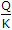B.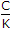, Q C.,D. C, K

Explanation:

Since the dimension of plates is scaled down by K, the area decreases by a factor of K2, the distance between plates decreases by a factor of K.

Therefore, capacitance changes to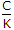.

The selectivity will not be affected.

27.

The hysteresis loss is

 A. proportional to frequency B. independent of frequency C. proportional to (frequency)2 D. proportional to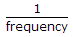Explanation:

No answer description available for this question. Let us discuss.

28.

If m, v, r are the mass, velocity and radius of orbit of electron and h is Planck's constant, quantum condition for electron's orbit is

 A.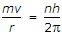B.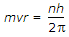C.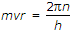D.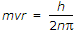Explanation:

For stable circular orbit angular momentum is equal to integer times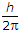.

29.

Assertion (A): For a magnetic material M = (μr - 1) H, where M is magnetic dipole moment/m3, H is field intensity A/m and μr is relative permeability.

Reason (R): μr can have any value from 0 to hundreds of thousands.

 A. Both A and R are true and R is correct explanation of A B. Both A and R are true but R is not correct explanation of A C. A is true but R is false D. A is false but R is true

Explanation:

μr is always more than 1. It cannot be 0 or less than 1.

30.

As regards ∈0 and ∈r (absolute and relative permittivity)

 A. both ∈0 and ∈r can be interpreted in terms of atomic properties of medium B. only ∈r can be interpreted in terms of atomic properties of medium C. only ∈0 can be interpreted in terms of atomic properties D. ∈r has no physical significance